# ML | V-Measure for Evaluating Clustering Performance

One of the primary disadvantages of any clustering technique is that it is difficult to evaluate its performance. To tackle this problem, the metric of V-Measure was developed.

The calculation of the V-Measure first requires the calculation of two terms:-

1. Homogenity: A perfectly homogeneous clustering is one where each cluster has data-points belonging to the same class label. Homogeneity describes the closeness of the clustering algorithm to this perfection.
2. Completeness: A perfectly complete clustering is one where all data-points belonging to the same class are clustered into the same cluster. Completeness describes the closeness of the clustering algorithm to this perfection.

Trivial Homogeneity: It is the case when the number of clusters is equal to the number of data points and each point is in exactly one cluster. It is the extreme case when homogeneity is highest while completeness is minimum.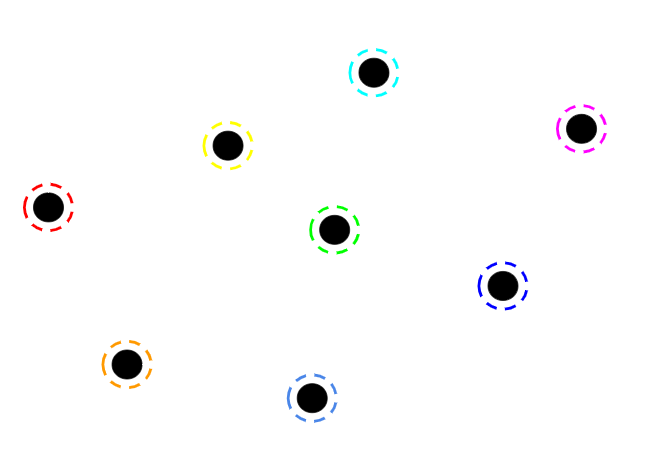Trivial Completeness: It is the case when all the data points are clustered into one cluster. It is the extreme case when homogeneity is minimum and completeness is maximum.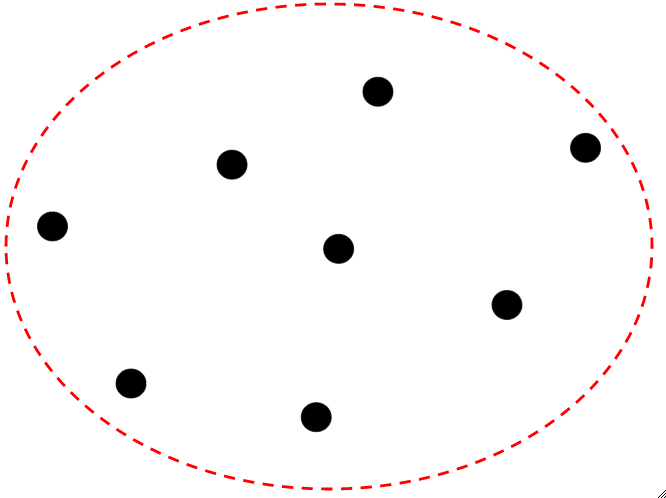Assume that each data point in the above diagrams is of the different class label for Trivial Homogeneity and Trivial Completeness.

Note: The term homogeneous is different from completeness in the sense that while talking about homogeneity, the base concept is of the respective cluster which we check whether in each cluster does each data point is of the same class label. While talking about completeness, the base concept is of the respective class label which we check whether data points of each class label is in the same cluster.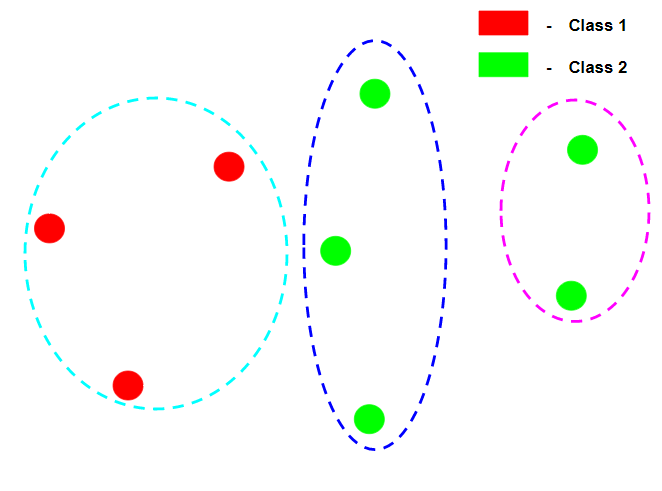In the above diagram, the clustering is perfectly homogeneous since in each cluster the data points of are of the same class label but it is not complete because not all data points of the same class label belong to the same class label.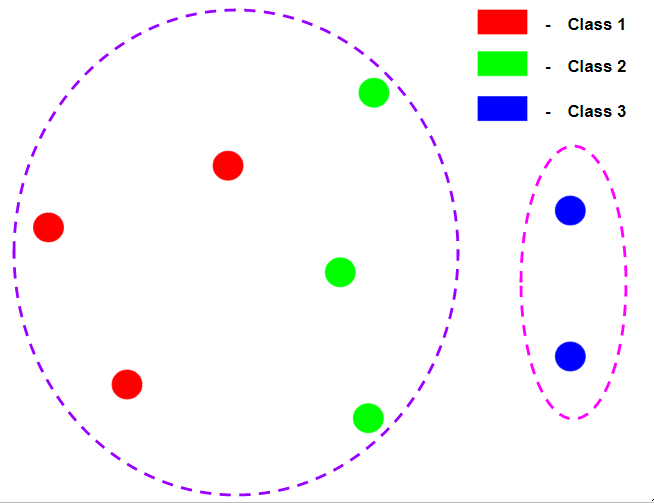In the above diagram, the clustering is perfectly complete because all data points of the same class label belong to the same cluster but it is not homogeneous because the 1st cluster contains data points of many class labels.

Let us assume that there are N data samples, C different class labels, K clusters andnumber of data-points belonging to the class c and cluster k. Then the homogeneity h is given by the following:-whereandThe completeness c is given by the following:-whereandThus the weighted V-Measureis given by the following:-The factorcan be adjusted to favour either the homogeneity or the completeness of the clustering algorithm.

The primary advantage of this evaluation metric is that it is independent of the number of class labels, the number of clusters, the size of the data and the clustering algorithm used and is a very reliable metric.

The following code will demonstrate how to compute the V-Measure of a clustering algorithm. The data used is the Detection of Credit Card Fraud which can be downloaded from Kaggle. The clustering algorithm used is the Variational Bayesian Inference for Gaussian Mixture Model.

Step 1: Importing the required libraries

 import pandas as pd  import matplotlib.pyplot as plt  from sklearn.cluster import KMeans  from sklearn.metrics import v_measure_score

 # Changing the working location to the location of the file  cd C:\Users\Dev\Desktop\Kaggle\Credit Card Fraud     # Loading the data  df = pd.read_csv('creditcard.csv')     # Separating the dependent and independent variables  y = df['Class']  X = df.drop('Class', axis = 1)     X.head()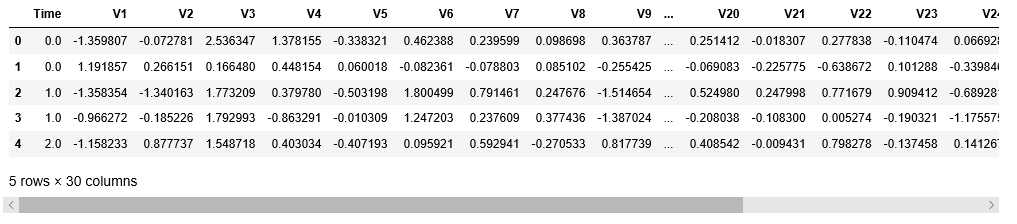Step 3: Building different clustering models and comparing their V-Measure scores

In this step, 5 different K-Means Clustering Models will be built with each model clustering the data into a different number of clusters.

 # List of V-Measure Scores for different models  v_scores = []     # List of different types of covariance parameters  N_Clusters = [2, 3, 4, 5, 6]

a) n_clusters = 2

 # Building the clustering model  kmeans2 = KMeans(n_clusters = 2)     # Training the clustering model  kmeans2.fit(X)     # Storing the predicted Clustering labels  labels2 = kmeans2.predict(X)     # Evaluating the performance  v_scores.append(v_measure_score(y, labels2))

b) n_clusters = 3

 # Building the clustering model  kmeans3 = KMeans(n_clusters = 3)     # Training the clustering model  kmeans3.fit(X)     # Storing the predicted Clustering labels  labels3 = kmeans3.predict(X)     # Evaluating the performance  v_scores.append(v_measure_score(y, labels3))

c) n_clusters = 4

 # Building the clustering model  kmeans4 = KMeans(n_clusters = 4)     # Training the clustering model  kmeans4.fit(X)     # Storing the predicted Clustering labels  labels4 = kmeans4.predict(X)     # Evaluating the performance  v_scores.append(v_measure_score(y, labels4))

d) n_clusters = 5

 # Building the clustering model  kmeans5 = KMeans(n_clusters = 5)     # Training the clustering model  kmeans5.fit(X)     # Storing the predicted Clustering labels  labels5 = kmeans5.predict(X)     # Evaluating the performance  v_scores.append(v_measure_score(y, labels5))

e) n_clusters = 6

 # Building the clustering model  kmeans6 = KMeans(n_clusters = 6)     # Training the clustering model  kmeans6.fit(X)     # Storing the predicted Clustering labels  labels6 = kmeans6.predict(X)     # Evaluating the performance  v_scores.append(v_measure_score(y, labels6))

Step 4: Visualizing the results and comparing the performances

 # Plotting a Bar Graph to compare the models  plt.bar(N_Clusters, v_scores)  plt.xlabel('Number of Clusters')  plt.ylabel('V-Measure Score')  plt.title('Comparison of different Clustering Models')  plt.show()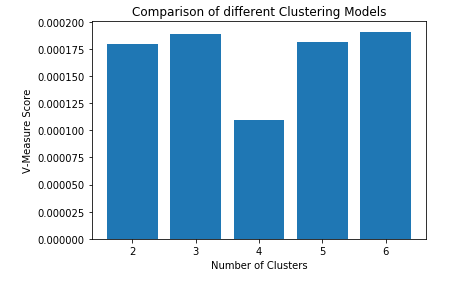My Personal Notes arrow_drop_upCheck out this Author's contributed articles.

If you like GeeksforGeeks and would like to contribute, you can also write an article using contribute.geeksforgeeks.org or mail your article to contribute@geeksforgeeks.org. See your article appearing on the GeeksforGeeks main page and help other Geeks.

Please Improve this article if you find anything incorrect by clicking on the "Improve Article" button below.

Improved By : shubham_singh

Article Tags :
Practice Tags :

Be the First to upvote.

Please write to us at contribute@geeksforgeeks.org to report any issue with the above content.